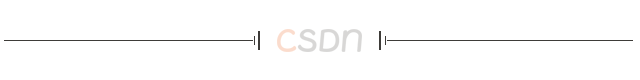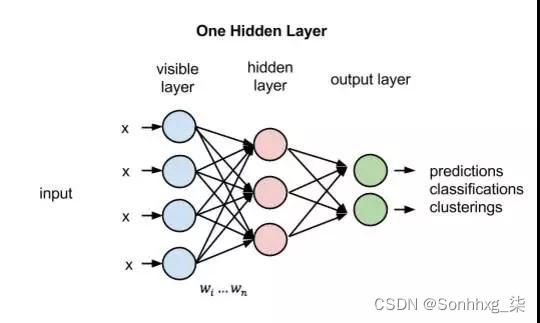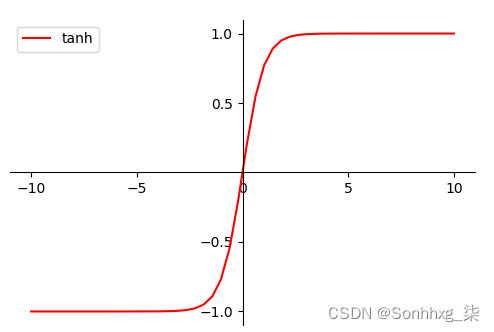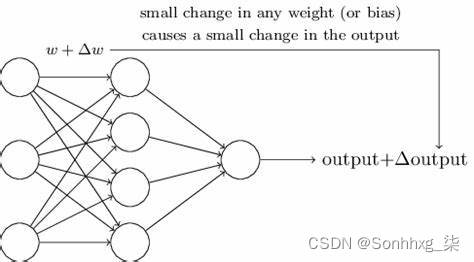# 【监督学习】多层感知器MLP（含代码实现）

🔎大家好，我是Sonhhxg_柒，希望你看完之后，能对你有所帮助，不足请指正！共同学习交流🔎

📝个人主页－Sonhhxg_柒的博客_CSDN博客 📃

🎁欢迎各位→点赞👍 + 收藏⭐️ + 留言📝​

📣系列专栏 – 机器学习【ML】 自然语言处理【NLP】  深度学习【DL】### 🖍foreword

✔说明⇢本人讲解主要包括Python、机器学习（ML）、深度学习（DL）、自然语言处理（NLP）等内容。

## 1、多层感知器MLPMLP多层感知器是一种前向结构的ANN人工神经网络, 多层感知器（MLP）能够处理非线性可分离的问题。

MLP概念：

MLP多层感知器(Multi-layerPerceptron)是一种前向结构人工神经网络ANN，映射一组输入向量到一组输出向量。MLP可以被看做是一个有向图，由多个节点层组成，每一层全连接到下一层。除了输入节点，每个节点都是一个带有非线性激活函数的神经元。使用BP反向传播算法的监督学习方法来训练MLP。MLP是感知器的推广，克服了感知器不能对线性不可分数据进行识别的弱点

MLP激活函数

MLP可使用任何形式的激活函数，譬如阶梯函数或逻辑乙形函数（logistic sigmoid function），但为了使用反向传播算法进行有效学习，激活函数必须限制为可微函数。由于具有良好可微性，很多乙形函数，尤其是双曲正切函数（Hyperbolictangent）及逻辑乙形函数，被采用为激活函数。MLP原理：

1）输入节点（Input Nodes）：也称为输入层，输入节点从外部世界提供信息，。在输入节点中，不进行任何的计算，仅向隐藏节点传递信息。

2）隐藏节点（Hidden Nodes）：隐藏节点和外部世界没有直接联系。这些节点进行计算，并将信息从输入节点传递到输出节点。隐藏节点也称为隐藏层。尽管一个前馈神经网络只有一个输入层和一个输出层，但网络里可以没有也可以有多个隐藏层。

3）输出节点（Output Nodes）：输出节点也称为输出层，负责计算，并从网络向外部世界传递信息。MLP多层感知器就是前馈神经网络的一个例子，除了一个输入层和一个输出层以外，至少包含有一个隐藏层。单层感知器只能学习线性函数，而多层感知器也可以学习非线性函数。

MLP训练过程：

MLP训练过程大致如下：

1）所有边的权重随机分配；

2）前向传播：利用训练集中所有样本的输入特征，作为输入层，对于所有训练数据集中的输入，人工神经网络都被激活，然后经过前向传播，得到输出值。

3）反向传播：利用输出值和样本值计算总误差，再利用反向传播来更新权重。

4）重复2)~3), 直到输出误差低于制定的标准。

MLP优点：

1）高度的并行处理；

2）高度的非线性全局作用；

3）良好的容错性；

4）具有联想记忆功能；

5）非常强的自适应、自学习功能。

MLP缺点：

1）网络的隐含节点个数选取非常难；

2）停止阈值、学习率、动量常数需要采用”trial-and-error”法，极其耗时；

3）学习速度慢；

4）容易陷入局部极值；

5）学习可能会不够充分。

MLP应用：

MLP在80年代的时候曾是相当流行的机器学习方法，拥有广泛的应用场景，譬如语音识别、图像识别、机器翻译等等，但自90年代以来，MLP遇到来自更为简单的支持向量机的强劲竞争。近来，由于深层学习的成功，MLP又重新得到了关注。

## 2、MLP的代码实现

``````from __future__ import print_function, division
import numpy as np
import math
from sklearn import datasets

from mlfromscratch.utils import train_test_split, to_categorical, normalize, accuracy_score, Plot
from mlfromscratch.deep_learning.activation_functions import Sigmoid, Softmax
from mlfromscratch.deep_learning.loss_functions import CrossEntropy

class MultilayerPerceptron():
"""Multilayer Perceptron classifier. A fully-connected neural network with one hidden layer.
Unrolled to display the whole forward and backward pass.
Parameters:
-----------
n_hidden: int:
The number of processing nodes (neurons) in the hidden layer.
n_iterations: float
The number of training iterations the algorithm will tune the weights for.
learning_rate: float
The step length that will be used when updating the weights.
"""
def __init__(self, n_hidden, n_iterations=3000, learning_rate=0.01):
self.n_hidden = n_hidden
self.n_iterations = n_iterations
self.learning_rate = learning_rate
self.hidden_activation = Sigmoid()
self.output_activation = Softmax()
self.loss = CrossEntropy()

def _initialize_weights(self, X, y):
n_samples, n_features = X.shape
_, n_outputs = y.shape
# Hidden layer
limit   = 1 / math.sqrt(n_features)
self.W  = np.random.uniform(-limit, limit, (n_features, self.n_hidden))
self.w0 = np.zeros((1, self.n_hidden))
# Output layer
limit   = 1 / math.sqrt(self.n_hidden)
self.V  = np.random.uniform(-limit, limit, (self.n_hidden, n_outputs))
self.v0 = np.zeros((1, n_outputs))

def fit(self, X, y):

self._initialize_weights(X, y)

for i in range(self.n_iterations):

# ..............
#  Forward Pass
# ..............

# HIDDEN LAYER
hidden_input = X.dot(self.W) + self.w0
hidden_output = self.hidden_activation(hidden_input)
# OUTPUT LAYER
output_layer_input = hidden_output.dot(self.V) + self.v0
y_pred = self.output_activation(output_layer_input)

# ...............
#  Backward Pass
# ...............

# OUTPUT LAYER
# Grad. w.r.t input of output layer
# HIDDEN LAYER
# Grad. w.r.t input of hidden layer

# Update weights (by gradient descent)
# Move against the gradient to minimize loss

# Use the trained model to predict labels of X
def predict(self, X):
# Forward pass:
hidden_input = X.dot(self.W) + self.w0
hidden_output = self.hidden_activation(hidden_input)
output_layer_input = hidden_output.dot(self.V) + self.v0
y_pred = self.output_activation(output_layer_input)
return y_pred

def main():
X = normalize(data.data)
y = data.target

# Convert the nominal y values to binary
y = to_categorical(y)

X_train, X_test, y_train, y_test = train_test_split(X, y, test_size=0.4, seed=1)

# MLP
clf = MultilayerPerceptron(n_hidden=16,
n_iterations=1000,
learning_rate=0.01)

clf.fit(X_train, y_train)
y_pred = np.argmax(clf.predict(X_test), axis=1)
y_test = np.argmax(y_test, axis=1)

accuracy = accuracy_score(y_test, y_pred)
print ("Accuracy:", accuracy)

# Reduce dimension to two using PCA and plot the results
Plot().plot_in_2d(X_test, y_pred, title="Multilayer Perceptron", accuracy=accuracy, legend_labels=np.unique(y))

if __name__ == "__main__":
main()``````

(0)